# Poisson Lie group

(diff) ← Older revision | Latest revision (diff) | Newer revision → (diff)

A Lie groupwith a Poisson structurewhich is compatible with the group multiplication, i.e., the multiplicationis a Poisson mapping, where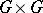is the product Poisson manifold (cf. Poisson algebra).

The corresponding infinitesimal object is a Lie bialgebra (see Quantum groups)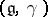, called the tangent Lie bialgebra of. Here,is the Lie algebra of the Lie groupand the linear mapping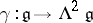is defined to be the linearization ofat the identity of the group; it is a Lie-algebra-cocycle with respect to the adjoint action (because of the compatibility condition) and a Lie cobracket, i.e., its transpose is a Lie bracket on the dualof. Conversely, any Lie bialgebra can be integrated to a unique (up to isomorphism) connected and simply connected Poisson Lie group.

To each Lie bialgebra structure onthere corresponds a Lie bialgebra structure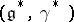on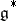, called the dual of, and a Lie bialgebra structure on, called the double of. Therefore, each Poisson Lie grouphas a dual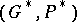, and a double, with underlying manifold. There are Poisson actions ofon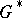, and ofon, called the dressing actions. The symplectic leaves of the Poisson manifold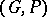are the orbits of the dressing action of.

An elementis called a solution of the classical (respectively, generalized) Yang–Baxter equation if the algebraic Schouten bracket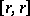ofwith itself vanishes (respectively, is ad-invariant). A solution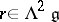of the classical Yang–Baxter equation is also called a triangular-matrix. A solution of the generalized Yang–Baxter equation defines a Lie bialgebra structure on, and a Poisson Lie structure on any Lie groupwith Lie algebra. In particular, a triangular-matrix defines both a left-invariant and a right-invariant Poisson structure onwhose difference is a Poisson Lie structure. An elementwith an invariant symmetric partis called a quasi-triangular-matrix if it satisfies, whereis its skew-symmetric part and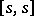is the ad-invariant element in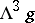, defined by, for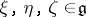. Thus, the skew-symmetric part of a quasi-triangular-matrix defines a Poisson Lie structure on. The equation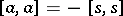, which reduces to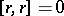when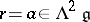, is variously called the classical Yang–Baxter equation, for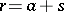, or the modified Yang–Baxter equation, for. It is usually written in the form.

Examples are: trivial Poisson Lie groups, where, with as dual Poisson Lie group the dual of the Lie algebra with its linear Poisson structure (also called the Kirillov–Kostant–Souriau Poisson structure), and with as double the cotangent bundle of; the simple Lie groups with the standard quasi-triangular-matrix; and the compact Lie groups with their Bruhat–Poisson structure.

It should be noted that the algebraic concept of a Lie bialgebra and the differential-geometric notion of a Poisson Lie group were first encountered as the classical limits of quantum objects participating in the quantum inverse scattering theory for integrable systems. (Thus, the classical Yang–Baxter equation is the classical limit of the quantum Yang–Baxter equation.) Conversely, the quantization problem is the problem of associating a quantum group to a given Poisson Lie group, i.e., of finding non-commutative deformations of its commutative algebra of functions, which is a Poisson–Hopf algebra (a Poisson algebra with a Hopf algebra structure such that the comultiplication is a morphism of Poisson algebras). Dually, quantum groups are also obtained as non-co-commutative deformations of the universal enveloping algebras of Lie bialgebras, which are co-commutative co-Poisson–Hopf algebras.

How to Cite This Entry:
Poisson Lie group. Encyclopedia of Mathematics. URL: http://encyclopediaofmath.org/index.php?title=Poisson_Lie_group&oldid=14191
This article was adapted from an original article by Y. Kosmann-Schwarzbach (originator), which appeared in Encyclopedia of Mathematics - ISBN 1402006098. See original article